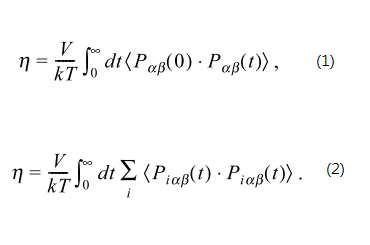# doing Green-Kubo correlation calculation on per-atom basis

Dear LAMMPS developers and users,

In the sample script of calculating viscosity via Green-Kubo formula, the pressure tensor is calculated as a system value:

http://lammps.sandia.gov/doc/Section_howto.html#howto_21

Yet, I would like to try another approach, namely, treat the pressure as per-atom value, do the auto-correlation, sum them up, and then integrate by time.

That being said, do equation 2 instead of equation 1 in the attached image file.

compute stress/atom gives the instantaneous pressure of each atom, but does fix ave/correlate treat arrays rather than single values?

Or is there any better way to perform this calculation?

Thank you for your attention. Any comment/suggestion is greatly appreciated.

LC Liu

ps. below is the reference talking about these two types of Green-Kubo formula.

http://www.journal.kcsnet.or.kr/main/j_search/j_abstract_view.htm?code=B030211&qpage=j_search&spage=b_bkcs&dpage=arFix ave/correlate does not work on vectors, but it sounds like you want
something akin to what the compute heat/flux command does for thermal
conductivity. It performs a summation of per-atom values that can
be then used by fix ave/correlate to extract kappa. See the compute
heat/flux doc page for details and an example script that uses it.

If you develop something similar for viscosity, it would be a nice command